# Rabin–Karp algorithm

The Rabin–Karp algorithm is a randomized algorithm for the string search problem that finds all probable matches for the needle in the haystack in linear time. Together with the use of a hash table, it can also find all probable matches for multiple needles in one haystack in linear time. Verifying all probable matches using the naive algorithm gives a Las Vegas algorithm, which requires up to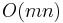$O(mn)$ time if the needle matches the haystack in many places or if many of the probable matches found are not true matches. Rabin–Karp can also be formulated as a Monte Carlo algorithm, which simply assumes all probable matches are correct, guaranteeing a linear runtime but with a small probability of false positives.

## Concept

Denote the needle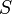$S$ and the haystack$T$. In the naive algorithm, we first test whether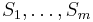$S_1, \ldots, S_m$ matches the substring at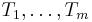$T_1, \ldots, T_m$. Then we try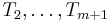$T_2, \ldots, T_{m+1}$, then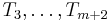$T_3, \ldots, T_{m+2}$, and so on; in other words, we compare the needle with all substrings of the same length in the haystack. Each equality comparison takes time proportional to the length of the needle. (In general, equality comparisons may take time proportional to the amount of data being compared. Hence, a CPU can often compare two machine words in one clock cycle, but will probably take 100 clock cycles to compare two 100-word strings.)

### Using hashing

To circumvent this problem, we can use a hash function, which takes a large amount of data as its argument and returns a relatively small amount of data, called the hash, which may be compared more quickly or easily. So we can rewrite the naive algorithm in the basic form:

s ← hash(S)
for i ∈ [0..n-m]
t ← hash(T[i+1 .. i+m])
if s = t
print "Probable match found at position " i


The equality comparison now only takes constant time, because it compares hash values instead of the actual strings. However, whereas inequality indicates a non-match, equality does not necessarily indicate a match, since hash functions cannot be injective; in this case we can only say we have a probable match. Thus, false negatives are impossible, but false positives are possible, and their probability is inversely proportional to the size of the range of the hash function.

### The rolling hash

The problem, however, is that a good hash function will always examine its entire input. Therefore, computing the hash function of a substring of the haystack takes time proportional to the length of that substring, so that the algorithm in its current form has best-case performance$O(mn)$ (computing the hash function once at each position of the haystack), which is as bad as the worst-case performance of the naive algorithm, and it also has a small probability of being wrong. Hence it is not clear that it is an improvement at all.

This problem is solved using a rolling hash. The key to the rolling hash is realizing that even though a good hash function has to examine all its input, there is significant overlap between the input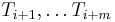$T_{i+1}, \ldots T_{i+m}$ and the next input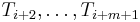$T_{i+2}, \ldots, T_{i+m+1}$. In fact, they share$m-2$ out of their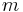$m$ characters! In light of this fact, perhaps we can design a hash function such that computing the hash of$T_{i+2}, \ldots, T_{i+m+1}$ does not require calling the function again, but can be done by examining the hash for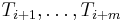$T_{i+1}, \ldots, T_{i+m}$ and somehow accounting for the two-character difference. This is a rolling hash.

One of the simplest rolling hashes is given by$H(a_0, a_1, \ldots, a_p) = a_0 + a_1 x + a_2 x^2 + \ldots + a_p x^p$, where$x$ is fixed and the polynomial evaluation is taken modulo a small, fixed base, usually the size of the machine word (which is a power of two), for the sake of efficiency. (To use this, we assign a non-negative integer to each character in the alphabet; often we simply use the ASCII code.) To see why this is useful, consider:$H_i = H(T_{i+1}, \ldots, T_{i+m}) = T_{i+1} + T_{i+2}x + \ldots + T_{i+m-1} x^{m-2} + T_{i+m}x^{m-1}$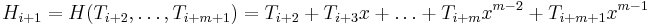$H_{i+1} = H(T_{i+2}, \ldots, T_{i+m+1}) = T_{i+2} + T_{i+3}x + \ldots + T_{i+m} x^{m-2} + T_{i+m+1}x^{m-1}$

How can we compute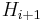$H_{i+1}$ given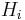$H_i$ and the text$T$? Looking closely, it becomes clear that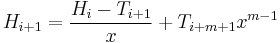$H_{i+1} = \frac{H_i-T_{i+1}}{x} + T_{i+m+1} x^{m-1}$.

## Implementation

We can now abstractly write the Rabin–Karp algorithm as follows:

s ← hash(S)
t ← hash(T[1..m])
if s = t
print "Probable match found at position 1"
for i ∈ [0..n-m-1]
t ← ((t-T[i+1])/x + T[i+m+1]·x^(m-1)) mod M
if s = t
print "Probable match found at position " i+2


To obtain a workable implementation, we use a few simple mathematical and computational tricks. The value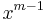$x^{m-1}$ (modulo M) can be computed efficiently via the square-and-multiply method. Division by$x$ is accomplished by multiplication by its modular inverse$x^{-1}$. This can be computed efficiently using the extended Euclidean algorithm, assuming$x$ is relatively prime with M (which is always true when$x$ is odd, since M is a power of two.) It often makes sense simply to precompute$x^{-1}$ and insert it as a literal into the code, to avoid having to implement the extended Euclidean algorithm.

### As a Las Vegas algorithm

s ← hash(S)
t ← hash(T[1..m])
if s = t
match ← true
for j ∈ [1..m]
if S[j] ≠ T[j]
match ← false
break
if match = true
print "Probable match found at position 1"
for i ∈ [0..n-m-1]
t ← ((t-T[i+1])/x + T[i+m+1]·x^(m-1)) mod M
if s = t
match ← true
for j ∈ [1..m]
if S[j] ≠ T[i+j+1]
match ← false
break
if match = true
print "Probable match found at position " i+2


### As a Monte Carlo algorithm

s ← hash(S)
t ← hash(T[1..m])
if s = t
print "Match found at position 1"
for i ∈ [0..n-m-1]
t ← ((t-T[i+1])/x + T[i+m+1]·x^(m-1)) mod M
if s = t
print "Match found at position " i+2


## Analysis

The precomputation steps (for$x^{-1}$ and$x^{m-1}$) take very little time (constant for the former, and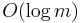$O(\log m)$ for the latter). Computing the hash value of the needle takes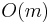$O(m)$ time, as does the initial hash computation for the haystack. "Rolling" the rolling hash takes constant time, and so does comparing the hashes. Thus the total time spent doing all these operations is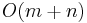$O(m+n)$ (linear).

However, the Las Vegas algorithm may takes$O(mn)$ time. This can happen, for example, when searching for AAAAAAA in AAAAAAAAAAAAA, and a match is found at every position; here, all the naive verification makes Rabin–Karp no better than the naive algorithm. However, if the needle is known to match the haystack only a few times, the result is much better; on average, one in every$M$ hash computations will result in a hash that matches that of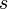$s$ (where$M$ is the modulus), giving, on the worst case, an extra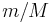$m/M$ operations, which is inconsequential. Note that in the analysis of the naive algorithm it was said that we could not rely on the average case to be random. With Rabin–Karp, however, even if there are many similar-looking substrings, they are unlikely to have matching hash values, and, conversely, those substrings that do have matching hash values are likely to be strikingly different in actual content, so that the inner verification loop will break relatively soon.

The Monte Carlo variation takes$O(m+n)$ time, but it has a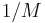$1/M$ probability of reporting a match at each position in$T$, regardless of whether or not an actual match occurs there. So the error rate is approximately$1/M$. If$M$ is made large enough (which is easy to accomplish with a library such as GMP) then the error rate can be made vanishingly small.

## Multiple needles

Suppose we are given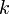$k$ needles, S, S, ..., S[k]. Then, by hashing all the needles using the same hash function and inserting them into a hash map, we can use the Rabin–Karp algorithm to find probable matches for all of them in the haystack in linear time; each time we compute the hash for a substring of the haystack, we look it up in the hash map to see whether it matches any of the needles' hashes.

H is a hash multimap
for i ∈ [1..k]
s ← hash(S[i])
H.insert(s,i)
t ← hash(T[1..m])
if H.find(t) ≠ ∅
for j ∈ H.find(t)
print "Probable match found at position 1 for needle " j
for i ∈ [0..n-m-1]
t ← ((t-T[i+1])/x + T[i+m+1]·x^(m-1)) mod M
if H.find(t) ≠ ∅
for j ∈ H.find(t)
print "Probable match found at position " i+2 " for needle " j